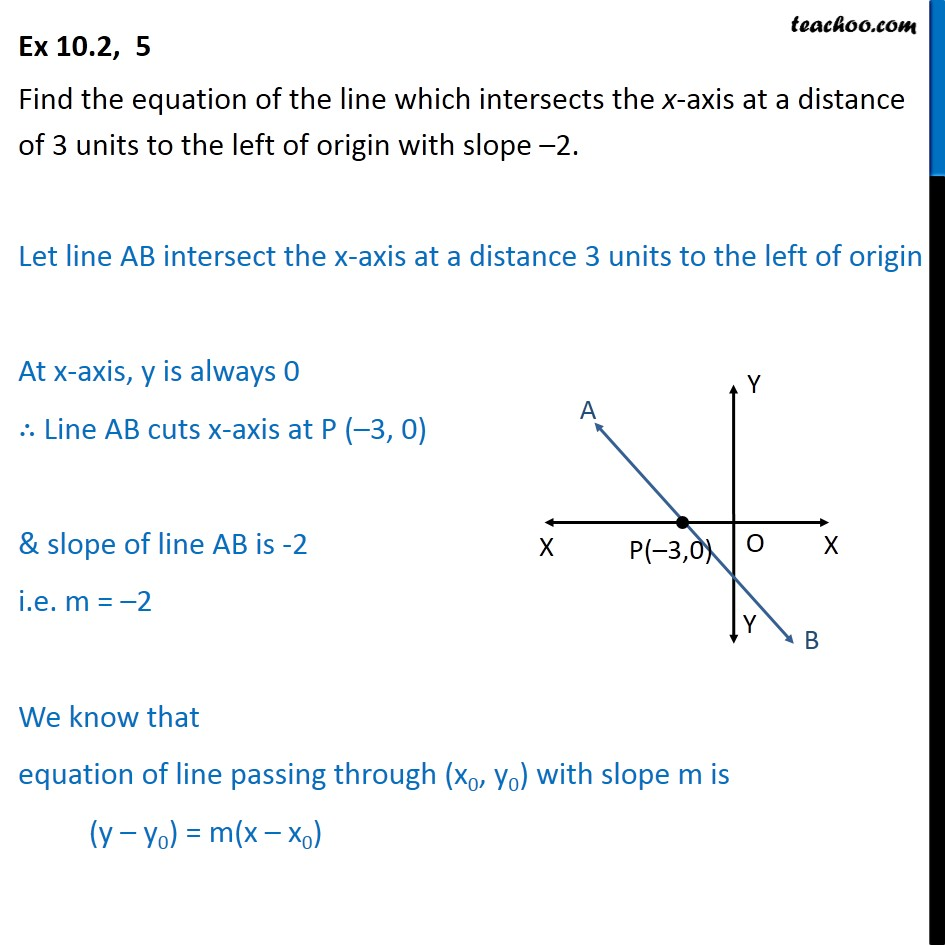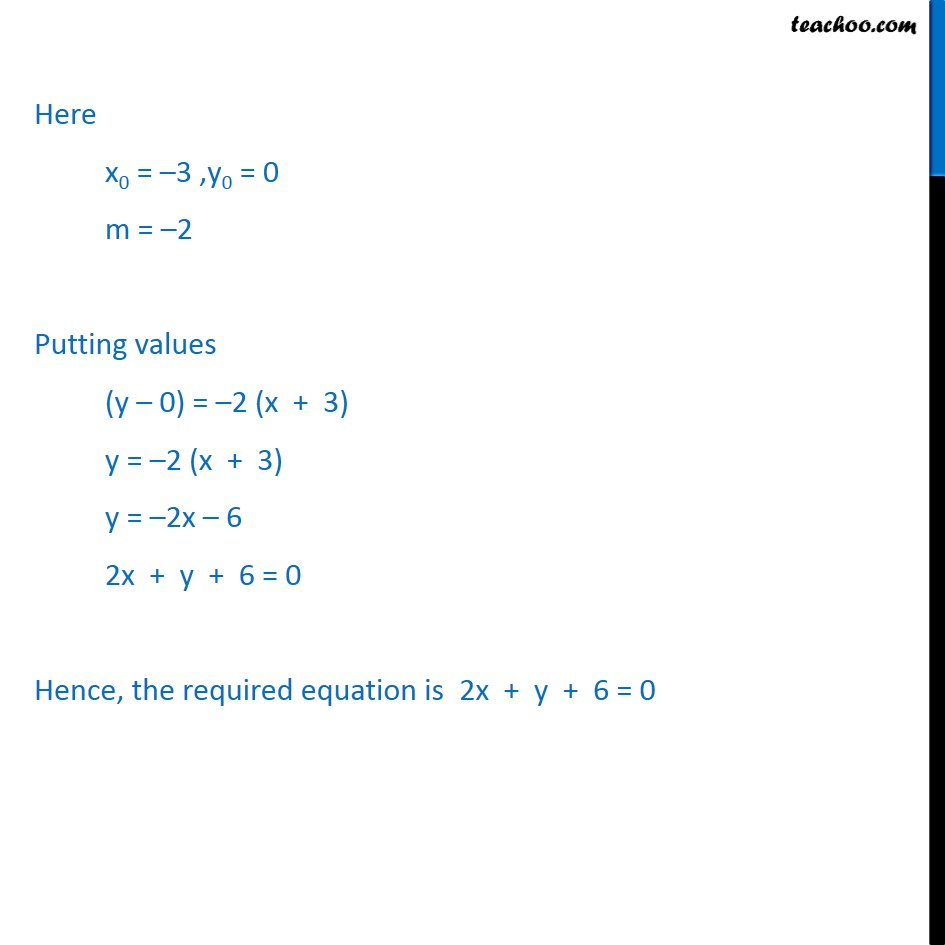Ex 10.2

Chapter 10 Class 11 Straight Lines (Term 1)
Serial order wise### Transcript

Ex 10.2, 5 Find the equation of the line which intersects the x-axis at a distance of 3 units to the left of origin with slope –2. Let line AB intersect the x-axis at a distance 3 units to the left of origin At x-axis, y is always 0 ∴ Line AB cuts x-axis at P (–3, 0) & slope of line AB is -2 i.e. m = –2 We know that equation of line passing through (x0, y0) with slope m is (y – y0) = m(x – x0) Here x0 = –3 ,y0 = 0 m = –2 Putting values (y – 0) = –2 (x + 3) y = –2 (x + 3) y = –2x – 6 2x + y + 6 = 0 Hence, the required equation is 2x + y + 6 = 0